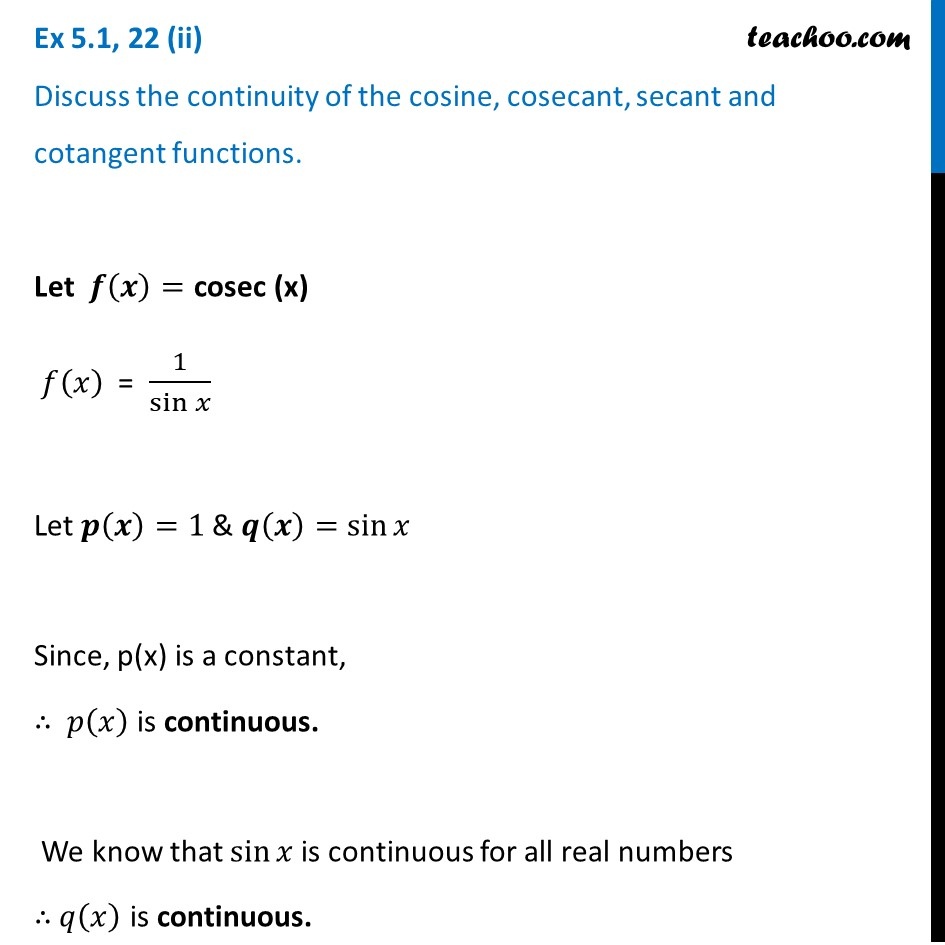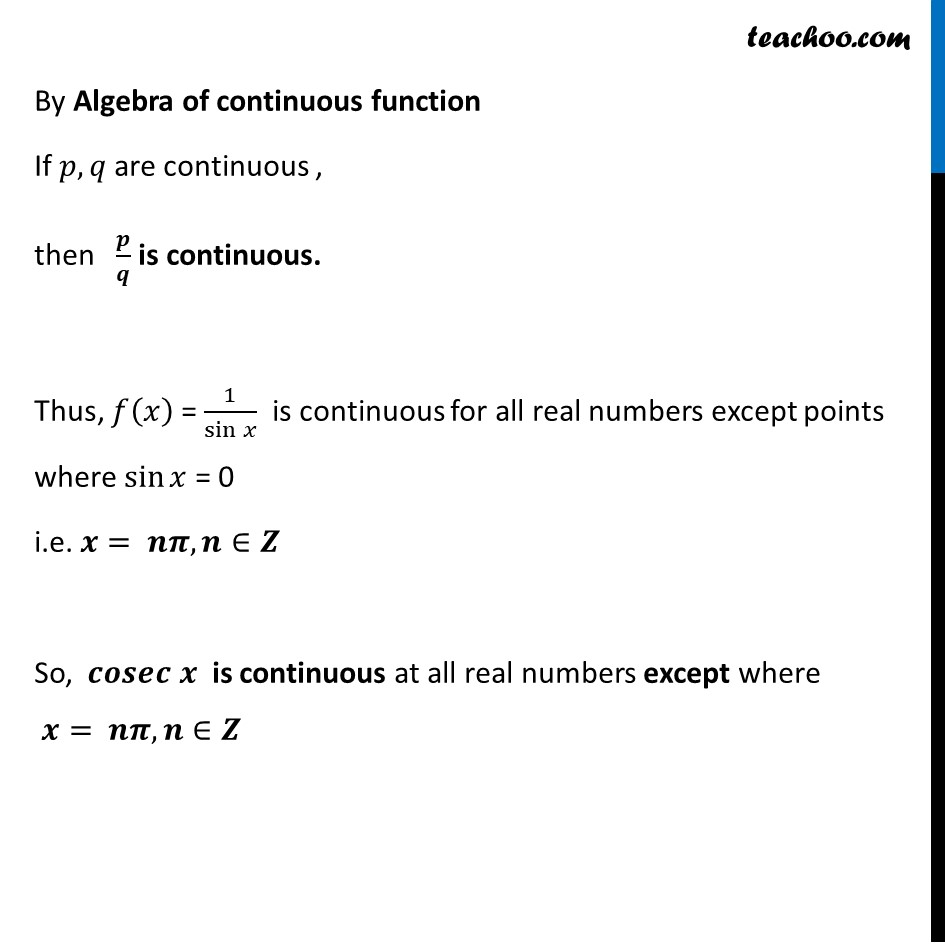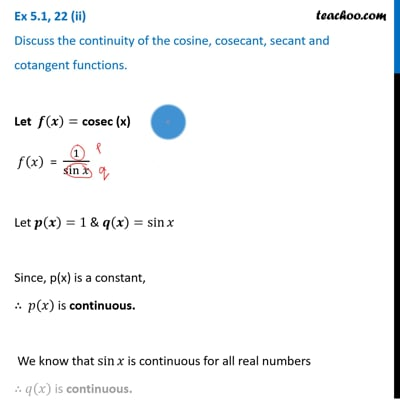Ex 5.1

Chapter 5 Class 12 Continuity and Differentiability
Serial order wiseThis video is only available for Teachoo black users

Introducing your new favourite teacher - Teachoo Black, at only ₹83 per month

### Transcript

Ex 5.1, 22 (ii) Discuss the continuity of the cosine, cosecant, secant and cotangent functions.Let 𝒇(𝒙)="cosec (x)" 𝑓(𝑥) = 1/sin⁡𝑥 Let 𝒑(𝒙)=1 & 𝒒(𝒙)=sin⁡𝑥 Since, p(x) is a constant, ∴ 𝑝(𝑥) is continuous. We know that sin⁡𝑥 is continuous for all real numbers ∴ 𝑞(𝑥) is continuous. By Algebra of continuous function If 𝑝, 𝑞 are continuous , then 𝒑/𝒒 is continuous. Thus, 𝑓(𝑥) = 1/sin⁡𝑥 is continuous for all real numbers except points where sin⁡𝑥 = 0 i.e. 𝒙= 𝒏𝝅, 𝒏∈𝒁 So, 𝒄𝒐𝒔𝒆𝒄 𝒙 is continuous at all real numbers except where 𝒙= 𝒏𝝅, 𝒏∈𝒁